# SAS/ETS Examples

## Analysis of Unobserved Component Models Using PROC UCM

Contents | SAS Program

# Overview

The UCM procedure analyzes and forecasts equally spaced univariate time series data using the Unobserved Components Model (UCM). A UCM decomposes a response series into components such as trend, seasonal, cycle, and the regression effects due to predictor series. These components capture the salient features of the series that are useful in explaining and predicting its behavior. The UCMs are also called Structural Models in the time series literature. This example illustrates the use of the UCM procedure by analyzing a yearly time series.

# A Series with Trend and a Cycle

The time series data analyzed in this example are annual age-adjusted melanoma incidences from the Connecticut Tumor Registry (Houghton, Flannery, and Viola 1980) for the years 1936 to 1972. The observations represent the number of melanoma cases per 100,000 people.

The following DATA step reads the data in and creates a date variable to label the measurements.

      data melanoma ;
input Incidences @@ ;
year = intnx('year','1jan1936'd,_n_-1) ;
format year year4. ;
label Incidences = 'Age Adjusted Incidences of Melanoma per 100,000';
datalines ;
0.9 0.8 0.8 1.3 1.4 1.2 1.7 1.8 1.6 1.5
1.5 2.0 2.5 2.7 2.9 2.5 3.1 2.4 2.2 2.9
2.5 2.6 3.2 3.8 4.2 3.9 3.7 3.3 3.7 3.9
4.1 3.8 4.7 4.4 4.8 4.8 4.8
;
run ;


Figure 1 shows a plot of the data.Figure 1: Melanoma Incidences Plot

To analyze this series, a UCM that contains a trend component, a cycle component, and an irregular component is appropriate. A time series yt that follows such a UCM can be formally described as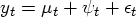whereis the trend component,is the cycle component, andis the error term. The error term is also called the irregular component, which is assumed to be a Gaussian white noise with variance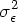. The trend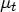is modeled as a stochastic component with slowly varying level and slope. Its evolution is described as follows:
The disturbances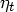and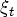are assumed to be independent. There are some interesting special cases of this trend model, obtained by setting one or both of the disturbance variances,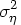and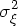, equal to zero. If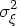is set equal to zero, then you get a linear trend model with fixed slope. If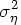is set to zero, then the resulting model usually has a smoother trend. If both the variances are set to zero, then the resulting model is the deterministic linear time trend,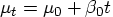.

The cycle componentis modeled as follows:
Hereis the damping factor, whereand the disturbances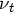andare independent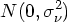variables. This results in a damped stochastic cycle that has time-varying amplitude and phase, and a fixed period equal to.

The parameters of this UCM are the different disturbance variances,,,, and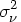; the damping factor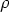; and the frequency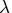.

The following syntax fits the UCM to the melanoma incidences series:

   proc ucm data = melanoma;
id year interval = year;
model Incidences ;
irregular ;
level ;
slope ;
cycle ;
run ;


Begin by specifying the input data set in the PROC statement. Second, use the ID statement in conjunction with the INTERVAL= statement to specify the time interval between observations. Note that the values of the ID variable are extrapolated for the forecast observations based on the values of the INTERVAL= option. Next, the MODEL statement is used to specify the dependent variable. If there are any predictors in the model, they are specified in the MODEL statement on the right-hand side of the equation. Finally, the IRREGULAR statement is used to specify the irregular component, the LEVEL and SLOPE statements are used to specify the trend component, and the CYCLE statement is used to specify the cycle component. Notice that different components in the model are specified by separate statements and that each component statement has a different set of options, which can be found in the SAS/ETS User's Guide. These options are useful for specifying additional details about that component. The following output from the UCM procedure in Figure 2 shows the parameter estimates for this model.

 Melanoma Incidences Plot

The UCM Procedure

Final Estimates of the Free Parameters
Component Parameter Estimate Approx
Std Error
t Value Approx
Pr > |t|
Irregular Error Variance 0.05706 0.01750 3.26 0.0011
Level Error Variance 7.328566E-9 4.70077E-6 0.00 0.9988
Slope Error Variance 8.71942E-11 5.61859E-8 0.00 0.9988
Cycle Damping Factor 0.96476 0.04857 19.86 <.0001
Cycle Period 9.68327 0.62859 15.40 <.0001
Cycle Error Variance 0.00302 0.0022975 1.31 0.1893

Figure 2: Parameter Estimates

The table shows that the disturbance variances for the level and slope components are highly insignificant. This suggests that a deterministic trend model may be more appropriate. The estimated period of the cycle is about 9.7 years. Interestingly, this is similar to another well-known cycle, the sun-spot activity cycle, which is known to have a period of 9 to 11 years. This provides some support for the claim that the melonama incidences are related to sun exposure. The estimate of the damping factor is 0.96, which is close to 1. This suggests that the periodic pattern of melanoma incidences does not diminish quickly.

The procedure outputs a variety of other statistics useful in model diagnostics, such as series forecasts and component estimates, which point toward the use of a deterministic trend model. You can construct this model with a fixed linear trend by holding the values of the level and slope disturbance variances fixed at zero. These types of modifications in the model specification are very easy to do in the UCM procedure. The following syntax illustrates some of this functionality.

   ods html ;
ods graphics on ;
proc ucm data = melanoma;
id year interval = year;
model Incidences ;
irregular ;
level variance=0 noest ;
slope variance=0 noest ;
cycle plot=smooth ;
estimate back=5 plot=(normal acf);
run ;
ods graphics off ;
ods html close ;


The ID, MODEL, and IRREGULAR statements appear as they did in the first model. In this model, however, you specify some specific options in the remaining component statements:

• In the LEVEL and SLOPE statements, the variances are set to zero to create a model with a fixed linear trend. A NOEST option is also included in these statements to fix the values of the model parameters.
• In the CYCLE statement, you can use the PLOT= option to plot the smoothed estimate of the cycle component.
• In the ESTIMATE statement, you are able to control the span of observations used in parameter estimation using the BACK= option. In this particular model, you set BACK=5 to specify a hold-out sample of five observations, which are omitted from the estimation. You can also plot the residual diagnostic plots using the PLOT= option.
• In the FORECAST statement, you use the LEAD= option to specify the number of periods to forecast beyond the historical period. In this case, you select to produce 10 multi-step forecasts. The BACK= option tells PROC UCM to begin the multi-step forecast five observations back from the end of the historical data. This corresponds with the beginning of the hold-out sample period specified by the BACK= option on the ESTIMATE statement. Thus a total of 10 multi-step forecasts are produced (five corresponding with the hold-out sample and five additional forecasts into the future). Finally, use the PLOT= option to generate the series decomposition plots.
• The ODS graphics on; statement invokes the ODS graphics system. The PLOT options on the CYCLE and FORECAST statements in the code cause ODS to produce high-resolution plots of the specified components. The ODS graphics off; statement turns off the graphics system. Note that the ODS Graphics System is experimental in SAS 9 and 9.1.

The parameter estimates for the deterministic trend model are shown in Figure 3:

The UCM Procedure

Final Estimates of the Free Parameters
Component Parameter Estimate Approx
Std Error
t Value Approx
Pr > |t|
Irregular Error Variance 0.05675 0.02387 2.38 0.0174
Cycle Damping Factor 0.94419 0.08743 10.80 <.0001
Cycle Period 9.76778 0.89263 10.94 <.0001
Cycle Error Variance 0.00590 0.0045948 1.28 0.1994

Figure 3: Parameter Estimates for Deterministic Trend Model

The procedure prints a variety of model diagnostic statistics by default (not shown). You can also request different residual plots. The model residual histogram and autocorrelation plots that follow in Figure 4 and Figure 5 do not show any serious violations of the model assumptions.Figure 4: Prediction Error Histogram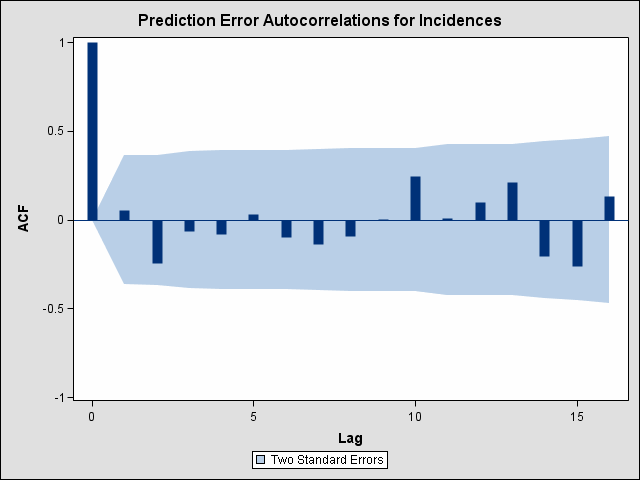Figure 5: Prediction Error Autocorrelations

The component plots in the model are useful for understanding the series' behavior and detecting structural breaks in the evolution of the series. The following plot in Figure 6 shows the smoothed estimate of the cycle component in the model.Figure 6: Smoothed Cycle Component

You can also plot and print the series forecasts. The 10-year ahead forecasted values and their confidence intervals are shown in the following table in Figure 7. Remember that five measurements from the hold-out sample are included.

Forecasts for Variable Incidences
Obs year Forecast Standard Error 95% Confidence Limits
33 1968 4.342356 0.30415 3.746235 4.938476
34 1969 4.550798 0.32420 3.915380 5.186216
35 1970 4.693234 0.33336 4.039858 5.346611
36 1971 4.763516 0.33408 4.108734 5.418299
37 1972 4.783619 0.33260 4.131739 5.435500
38 1973 4.792227 0.33172 4.142069 5.442386
39 1974 4.828202 0.33070 4.180042 5.476362
40 1975 4.915774 0.33029 4.268425 5.563122
41 1976 5.056911 0.33408 4.402118 5.711704
42 1977 5.232987 0.34403 4.558710 5.907264

Figure 7: Forecasts for Variable Incidences

The observations beyond the hold-out sample indicate that four to five incidences of melanoma per 100,000 people can be expected in the next five years.

You can also obtain a model-based "decomposition" of the series that shows the incremental effects of adding together different components that are present in the model. The following trend and trend plus cycle plots in Figure 8 and Figure 9 show such a decomposition in the current example.Figure 8: Smoothed Trend Estimate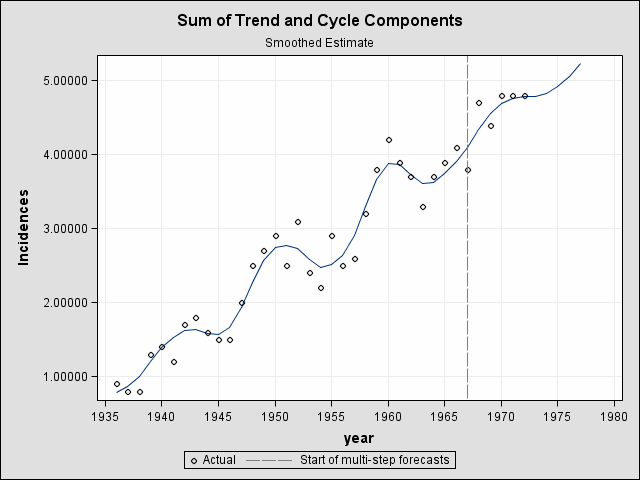Figure 9: Sum of Trend and Cycle Components

The plot shows that the melanoma incidences are expected to increase over the next decade with some cyclical fluctuations.

# References

Houghton, A. N., Flannery, J., and Viola, V. M. (1980), "Malignant Melanoma in Connecticut and Denmark," International Journal of Cancer, 25, 95-114.

SAS Institute Inc. (2002), SAS/ETS User's Guide, Version 9, Cary, NC: SAS Institute Inc.